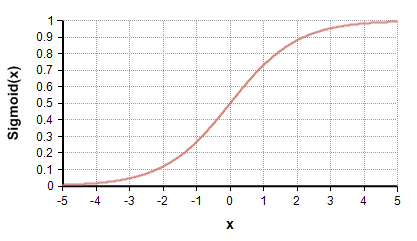# Sigmoid

## Sigmoid(x)

The Sigmoid function is

$\displaystyle{ Sigmoid(x) = {1\over{1+\exp(-x)}} }$The Sigmoid function goes by several other names including the logistic function, the inverse logit function, and the expit function.

There are other functions that are also sigmoidal in shape, most notably the ArcTan and Tanh functions. These other sigmoidal fucntions differ in their asymptotic values. The Sigmoid(x) function goes to 0 as «x» goes to $\displaystyle{ -\infty }$ and to 1 as «x» goes to $\displaystyle{ +\infty }$.

The inverse of the Sigmoid function is the Logit function (aka log-odds function). Hence, Sigmoid returns the probability value that corresponds to a given log-odds.

## Library

Sigmoid(Sum(c*x, K))